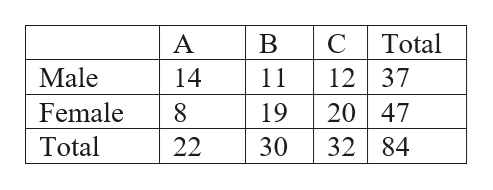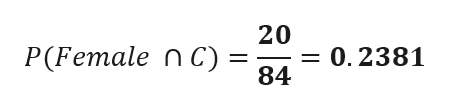Score on last attempt:   0.3 out of 1 (parts:  0.25/0.25,  0/0.25,  0/0.25,  0/0.25)Score in gradebook:   0.3 out of 1 (parts:  0.25/0.25,  0/0.25,  0/0.25,  0/0.25)Try another similar questionQuestion with last attempt is displayed for your review onlyGiving a test to a group of students, the grades and gender are summarized below ABCTotalMale14111237Female8192047Total22303284Write all solutions as a decimals to the fourth. If one student is chosen at random,Find the probability that the student was male:    Find the probability that the student was female AND got a "C":    Find the probability that the student was male OR got an "C":    If one student is chosen at random, find the probability that the student got a 'A' GIVEN they are female:

Question

Score on last attempt:   0.3 out of 1 (parts:  0.25/0.25,  0/0.25,  0/0.25,  0/0.25)

Score in gradebook:   0.3 out of 1 (parts:  0.25/0.25,  0/0.25,  0/0.25,  0/0.25)

Try another similar question

Question with last attempt is displayed for your review only

Giving a test to a group of students, the grades and gender are summarized below

 A B C Total Male 14 11 12 37 Female 8 19 20 47 Total 22 30 32 84

Write all solutions as a decimals to the fourth. If one student is chosen at random,

Find the probability that the student was male:

Find the probability that the student was female AND got a "C":

Find the probability that the student was male OR got an "C":

If one student is chosen at random, find the probability that the student got a 'A' GIVEN they are female:
Step 1

Given Datahelp_outlineImage TranscriptioncloseTotal A 12 37 20 47 32 84 Male 14 11 Female 8 19 Total 22 30 fullscreen
Step 2

a) probability that the student was male

Step 3

b)  probability that the stud...help_outlineImage Transcriptionclose20 = 0.2381 84 P(Female n C) fullscreen

Want to see the full answer?

See Solution

Want to see this answer and more?

Our solutions are written by experts, many with advanced degrees, and available 24/7

See Solution
Tagged in

Basic Probability